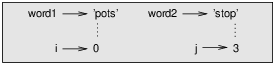# 8.11: Debugging

$$\newcommand{\vecs}{\overset { \rightharpoonup} {\mathbf{#1}} }$$ $$\newcommand{\vecd}{\overset{-\!-\!\rightharpoonup}{\vphantom{a}\smash {#1}}}$$$$\newcommand{\id}{\mathrm{id}}$$ $$\newcommand{\Span}{\mathrm{span}}$$ $$\newcommand{\kernel}{\mathrm{null}\,}$$ $$\newcommand{\range}{\mathrm{range}\,}$$ $$\newcommand{\RealPart}{\mathrm{Re}}$$ $$\newcommand{\ImaginaryPart}{\mathrm{Im}}$$ $$\newcommand{\Argument}{\mathrm{Arg}}$$ $$\newcommand{\norm}{\| #1 \|}$$ $$\newcommand{\inner}{\langle #1, #2 \rangle}$$ $$\newcommand{\Span}{\mathrm{span}}$$ $$\newcommand{\id}{\mathrm{id}}$$ $$\newcommand{\Span}{\mathrm{span}}$$ $$\newcommand{\kernel}{\mathrm{null}\,}$$ $$\newcommand{\range}{\mathrm{range}\,}$$ $$\newcommand{\RealPart}{\mathrm{Re}}$$ $$\newcommand{\ImaginaryPart}{\mathrm{Im}}$$ $$\newcommand{\Argument}{\mathrm{Arg}}$$ $$\newcommand{\norm}{\| #1 \|}$$ $$\newcommand{\inner}{\langle #1, #2 \rangle}$$ $$\newcommand{\Span}{\mathrm{span}}$$$$\newcommand{\AA}{\unicode[.8,0]{x212B}}$$

When you use indices to traverse the values in a sequence, it is tricky to get the beginning and end of the traversal right. Here is a function that is supposed to compare two words and return True if one of the words is the reverse of the other, but it contains two errors:

def is_reverse(word1, word2):
if len(word1) != len(word2):
return False

i = 0
j = len(word2)

while j > 0:
if word1[i] != word2[j]:
return False
i = i+1
j = j-1

return True


The first if statement checks whether the words are the same length. If not, we can return False immediately. Otherwise, for the rest of the function, we can assume that the words are the same length. This is an example of the guardian pattern in Section 6.8.

i and j are indices: i traverses word1 forward while j traverses word2 backward. If we find two letters that don’t match, we can return False immediately. If we get through the whole loop and all the letters match, we return True.

If we test this function with the words “pots” and “stop”, we expect the return value True, but we get an IndexError:

>>> is_reverse('pots', 'stop')
...
File "reverse.py", line 15, in is_reverse
if word1[i] != word2[j]:
IndexError: string index out of range


For debugging this kind of error, my first move is to print the values of the indices immediately before the line where the error appears.

    while j > 0:
print(i, j)        # print here

if word1[i] != word2[j]:
return False
i = i+1
j = j-1


>>> is_reverse('pots', 'stop')
0 4
...
IndexError: string index out of range


The first time through the loop, the value of j is 4, which is out of range for the string 'pots'. The index of the last character is 3, so the initial value for j should be len(word2)-1.

If I fix that error and run the program again, I get:

>>> is_reverse('pots', 'stop')
0 3
1 2
2 1
True


This time we get the right answer, but it looks like the loop only ran three times, which is suspicious. To get a better idea of what is happening, it is useful to draw a state diagram. During the first iteration, the frame for is_reverse is shown in Figure $$\PageIndex{1}$$.Figure $$\PageIndex{1}$$: State diagram.

I took some license by arranging the variables in the frame and adding dotted lines to show that the values of i and j indicate characters in word1 and word2.

Starting with this diagram, run the program on paper, changing the values of i and j during each iteration. Find and fix the second error in this function.

This page titled 8.11: Debugging is shared under a CC BY-NC 3.0 license and was authored, remixed, and/or curated by Allen B. Downey (Green Tea Press) .